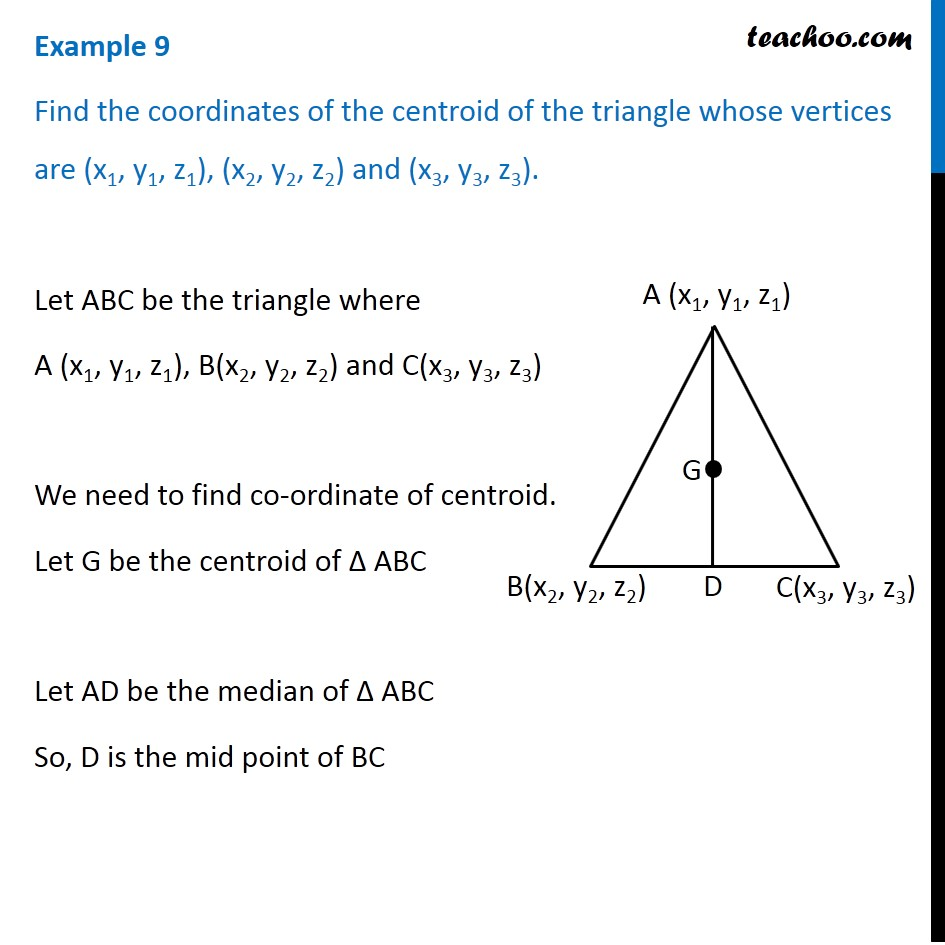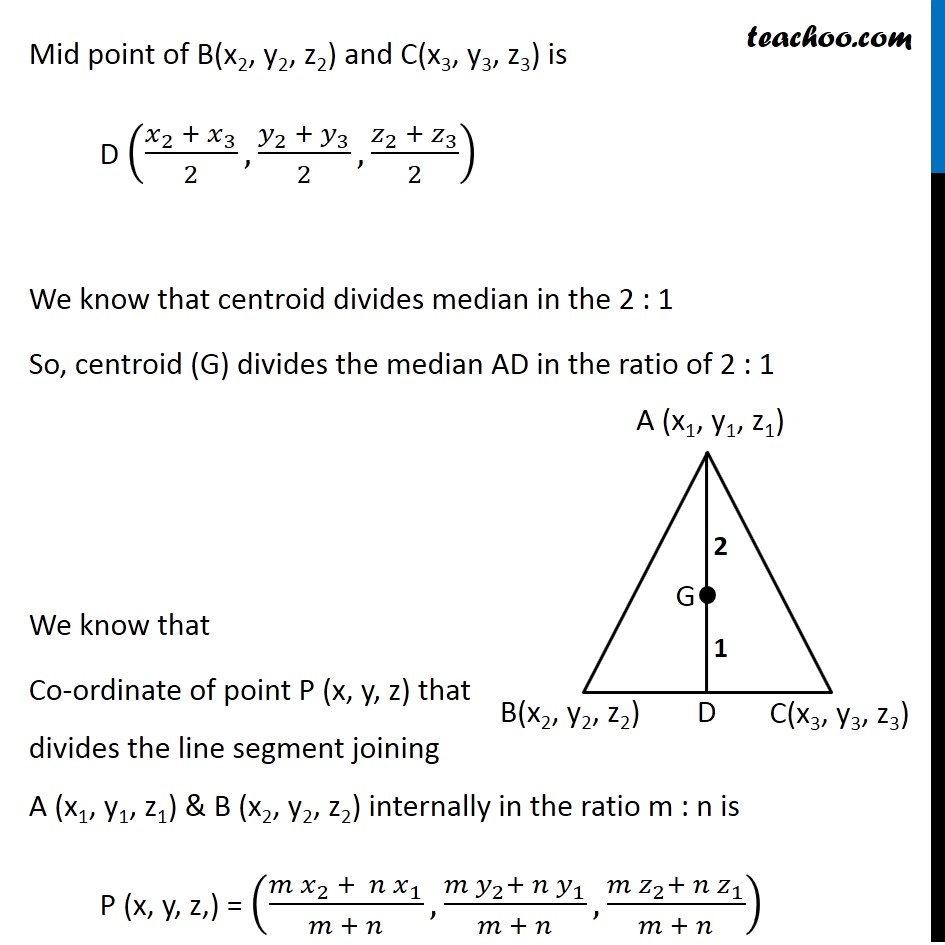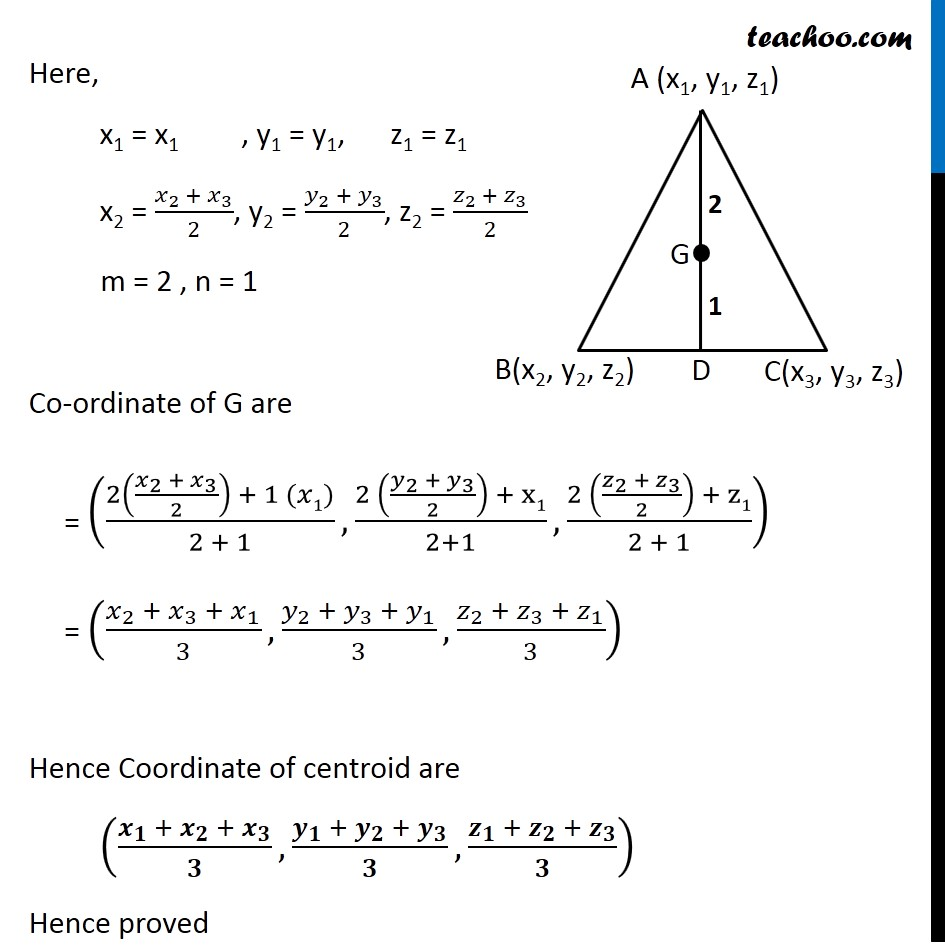Examples

Chapter 11 Class 11 - Intro to Three Dimensional Geometry
Serial order wiseLearn in your speed, with individual attention - Teachoo Maths 1-on-1 Class

### Transcript

Question 3 Find the coordinates of the centroid of the triangle whose vertices are (x1, y1, z1), (x2, y2, z2) and (x3, y3, z3). Let ABC be the triangle where A (x1, y1, z1), B(x2, y2, z2) and C(x3, y3, z3) We need to find co-ordinate of centroid. Let G be the centroid of ∆ ABC Let AD be the median of Δ ABC So, D is the mid point of BC Mid point of B(x2, y2, z2) and C(x3, y3, z3) is D ((𝑥_2 + 𝑥_3)/2,(𝑦_2 + 𝑦_3)/2,(𝑧_2 + 𝑧_3)/2) We know that centroid divides median in the 2 : 1 So, centroid (G) divides the median AD in the ratio of 2 : 1 We know that Co-ordinate of point P (x, y, z) that divides the line segment joining A (x1, y1, z1) & B (x2, y2, z2) internally in the ratio m : n is P (x, y, z,) = ((〖𝑚 𝑥〗_2 + 〖 𝑛 𝑥〗_1)/(𝑚 + 𝑛),(〖𝑚 𝑦〗_2+〖 𝑛 𝑦〗_1)/(𝑚 + 𝑛),(〖𝑚 𝑧〗_2+〖 𝑛 𝑧〗_1)/(𝑚 + 𝑛)) Here, x1 = x1 , y1 = y1, z1 = z1 x2 = (𝑥_(2 )+ 𝑥_3)/2, y2 = (𝑦_2 +〖 𝑦〗_3)/2, z2 = (𝑧_(2 )+ 𝑧_3)/2 m = 2 , n = 1 Co-ordinate of G are = ((2((𝑥_(2 )+ 𝑥_3)/2) + 1 (𝑥1))/(2 + 1),(2 ((𝑦_2 +〖 𝑦〗_3)/2) + x1)/(2+1),(2 ((𝑧_(2 )+ 𝑧_3)/2) + z1)/(2 + 1)) = ((𝑥_2 + 𝑥_3 + 𝑥_1)/3,(𝑦_2 + 𝑦_3 + 𝑦_1)/3,(𝑧_2 + 𝑧_3 + 𝑧_1)/3) Hence Coordinate of centroid are ((𝒙_𝟏 + 𝒙_𝟐 + 𝒙_𝟑)/𝟑,(𝒚_𝟏 + 𝒚_𝟐 + 𝒚_𝟑)/𝟑,(𝒛_𝟏 + 𝒛_𝟐 + 𝒛_𝟑)/𝟑) Hence proved